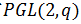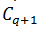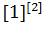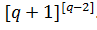Global Dimensional Mathematics

Olivier Denis

Journal of Advances in Mathematics and Computer Science, Page 1-10
DOI: 10.9734/jamcs/2021/v36i730378

Here we build the fundamentals of global dimensional mathematics in order to build the new basis of the new theoretical scientific paradigm.

Research on the foundations of mathematics covering the definition of fundamental mathematical concepts such as point, line, direction, dimension, addition, multiplication, division, zeros, infinities, limits, factorization, integers and prime numbers, were carried out and further still the resolution of the Goldbach conjecture is now effective.

New original fundamental mathematical notions are established building the core of global dimensional mathematics based on the decomposition of integers into an addition of prime numbers terms and on fundamental geometric concepts such as the concept dimension based on the notion of direction and point, as well as research on set theory and work on the notion of limits and infinity.

The termization as a decomposition of integers into an addition of prime number terms by a python program, breaks the unique factorization theorem, the fundamental theorem of arithmetic while the geometric notions developed break Euclidean geometry leading to a new mathematical framework, geometry, topology and metrics leading to a total change of theoretical scientific paradigm.

Global dimensional mathematics forms the basis for the construction of the new scientific paradigm of the 21st century and beyond, opening up a still unknown perspective on the world of science in general.

Action of PGL(2,q) on the Cosets of the Centralizer of an Eliptic Element

Journal of Advances in Mathematics and Computer Science, Page 11-20
DOI: 10.9734/jamcs/2021/v36i730379

Most researchers consider the action of projective general group on the cosets of its maximal subgroups leaving out non-maximal subgroups. In this paper, we consider the action ofcentralizer of an elliptic element which is a non maximal subgroup. In particular, we determine the subdegrees, rank and properties of the suborbital graphs of the action. We achieve this through the application of the action of a group by conjugation. We have proved that the rank is q  and the subdegrees areand.

Possibility to Construct a Machine for Primality Testing of Numbers

Takaaki Musha

Journal of Advances in Mathematics and Computer Science, Page 21-29
DOI: 10.9734/jamcs/2021/v36i730380

Like the optical prism to break white light up into its constituent spectral colors, the machine to show a prime as a single spectrum is proposed. From the theoretical analysis, it can be shown that the machine to recognize the prime number as a single spectrum can be realized by using the correlation function of Riemann zeta function. Moreover, this method can be used for a factorization of the integer consisted of two primes.

Multimodal Video Sentiment Analysis Using Audio and Text Data

Yanyan Wang

Journal of Advances in Mathematics and Computer Science, Page 30-37
DOI: 10.9734/jamcs/2021/v36i730381

Nowadays, video sharing websites are becoming more and more popular, such as YouTube, Tiktok. A good way to analyze a video’s sentiment would greatly improve the user experience and would help with designing better ranking and recommendation systems [1,2]. In this project, we used both acoustic information of a video to predict its sentiment levels. For audio data, we leverage transfer learning technique and use a pre-trained VGGish model as a features extractor to analyze abstract audio embeddings . We then used MOSI dataset  to further fine-tune the VGGish model and achieved a test accuracy of 90% for binary classification. For text data, we compared traditional bag-of-word model to LSTM model. We found that LSTM model with word2vec outperformed bag-of-word model and achieved a test accuracy of 84% for binary classification.

Application of Scalar Type Operators to Decomposability

Journal of Advances in Mathematics and Computer Science, Page 38-44
DOI: 10.9734/jamcs/2021/v36i730382

In this paper, we give some application of scalar type operators to Decomposibility. In particular, we show that if H is of (α, α + 1) type R and that it generates a strongly continuous group on a Banach space, then its resolvent is Decomposable hence scalar type.

The Energy of Conjugate Graph of Some Finite Metabelian Groups of Order Less Than 24

M. S. Mahmud, A. A. Malle, C. Chibuisi, M. Z. Idris

Journal of Advances in Mathematics and Computer Science, Page 45-51
DOI: 10.9734/jamcs/2021/v36i730383

The conjugacy classes of the Metabelian group G, plays an important role in defining the conjugate graph, whose vertices are non-central elements of G, and two vertices are connected if and only if they are conjugate. The constructions of conjugate graphs of all non abelian metabelian groups of order less than 24 are the basis for this paper. And the obtained results are then used to calculate the energy of the aforementioned group. This is aided by specialized programming software (maple).

On the Characteristics and Application of Inverse Power Pranav Distribution

K. C. Nwankwo, I. S. Onyeagu, C. H. Nwankwo, G. A. Osuji, C. K. Onyekwere

Journal of Advances in Mathematics and Computer Science, Page 52-65
DOI: 10.9734/jamcs/2021/v36i730384

In this article, we study the mathematical characteristics of the inverse power Pranav distribution. The proposed distribution has three special cases namely Pranav, inverse Pranav and inverse power Pranav distributions. In addition with the basic properties of the distribution, the maximum likelihood method was employed in computing the parameters of the distribution. The 95% confidence interval was estimated for each of the parameters and finally, the distribution was applied to 128 bladder cancer patients to illustrate its applicability, and compared to Pranav distribution, inverse power Lindley distribution and inverse Ishita distribution. However, the inverse power Pranav distribution proved superiority over the competing models.

Mathematical Modeling of Treatment Effect on Tumor Growth and Blood Flow through a Channel with Magnetic Field

K. W. Bunonyo, C. U. Amadi

Journal of Advances in Mathematics and Computer Science, Page 66-79
DOI: 10.9734/jamcs/2021/v36i730385

In this research, we investigated the effect of tumor growth on blood flow through a micro channel by formulated the governing model with the assumption that blood is an incompressible, eclectrially conducting fluid which flow is caused by the pumping action of the heart and suction. The governing model was scaled using some dimensionless variables and the region of the tumor was obtained from Dominguez  which was incorporated in our model. The model is further reduced to an ordinary differential equation using a perturbation condition. However, the ordinary differential equation was solved using method of undermined coefficients, and the constants coefficients obtained via matrix method. Furthermore, the simulation to study the effect of the pertinent parameters was done suing computation software called Mathematica. It is seen in our investigation that the entering parameters such as magnetic field parameter, the Reynolds number, womersley number, oscillatory frequency parameter, and permeability parameter affect the blood velocity profile in decreasing and increasing fashion.